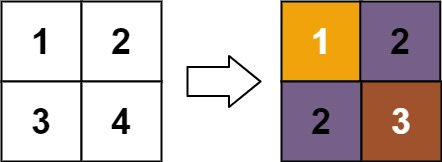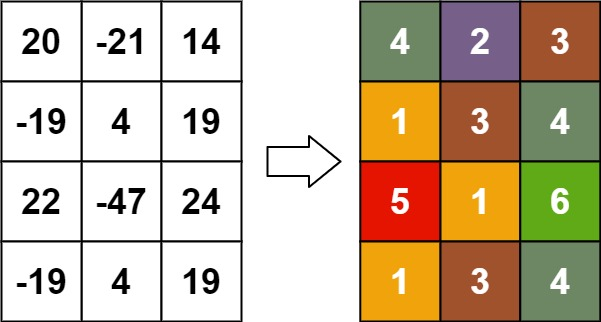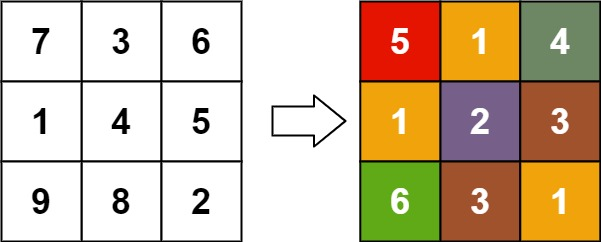Formatted question description: https://leetcode.ca/all/1632.html

# 1632. Rank Transform of a Matrix (Hard)

Given an m x n matrix, return a new matrix answer where answer[row][col] is the rank of matrix[row][col].

The rank is an integer that represents how large an element is compared to other elements. It is calculated using the following rules:

• The rank is an integer starting from 1.
• If two elements p and q are in the same row or column, then:
• If p < q then rank(p) < rank(q)
• If p == q then rank(p) == rank(q)
• If p > q then rank(p) > rank(q)
• The rank should be as small as possible.

It is guaranteed that answer is unique under the given rules.

Example 1:Input: matrix = [[1,2],[3,4]]
Output: [[1,2],[2,3]]
Explanation:
The rank of matrix is 1 because it is the smallest integer in its row and column.
The rank of matrix is 2 because matrix > matrix and matrix is rank 1.
The rank of matrix is 2 because matrix > matrix and matrix is rank 1.
The rank of matrix is 3 because matrix > matrix, matrix > matrix, and both matrix and matrix are rank 2.


Example 2:Input: matrix = [[7,7],[7,7]]
Output: [[1,1],[1,1]]


Example 3:Input: matrix = [[20,-21,14],[-19,4,19],[22,-47,24],[-19,4,19]]
Output: [[4,2,3],[1,3,4],[5,1,6],[1,3,4]]


Example 4:Input: matrix = [[7,3,6],[1,4,5],[9,8,2]]
Output: [[5,1,4],[1,2,3],[6,3,1]]


Constraints:

• m == matrix.length
• n == matrix[i].length
• 1 <= m, n <= 500
• -109 <= matrix[row][col] <= 109

Related Topics:
Greedy, Union Find

Similar Questions:

## Solution 1. Union Find

Intuition: Sort the numbers in ascending order. Visit them one by one. Each number should have a rank that is greater than those smaller numbers in the same row/column that are already assigned ranks.

The tricky part is how to handle those numbers of the same value. The numbers that have the same value and are in the same row/column should use the same rank, and the rank is the greatest one of those ranks if we check them individually.

To group those numbers, we can use Union Find.

If two numbers in the same row share the same value, we should connect the two column indexes.

If two numbers in the same column share the same value, we should connect the two row indexes.

Basically we are regarding the row and column indexes as vertices in a graph, and the numbers in the matrix as edge.

Time complexity:

For pushing into the map, in the worst case where all the numbers are different, the map will have MN distinct values, so it’s O(MNlog(MN)).

For each of the loops with Union Find, the time complexity is O(K) where K is the count of the pos array. So the overall time complexity of the Union Find loop is O(MN).

In sum, the time complexity is O(MNlog(MN)).

// OJ: https://leetcode.com/problems/rank-transform-of-a-matrix/

// Time: O(MNlog(MN))
// Space: O(MN)
// Ref: https://leetcode.com/problems/rank-transform-of-a-matrix/discuss/909142/Python-Union-Find
class UnionFind {
vector<int> id;
public:
UnionFind(int n) : id(n) {
iota(begin(id), end(id), 0);
}
void connect(int a, int b) {
int x = find(a), y = find(b);
if (x == y) return;
id[x] = y;
}
int find(int a) {
return id[a] == a ? a : (id[a] = find(id[a]));
}
};
class Solution {
public:
vector<vector<int>> matrixRankTransform(vector<vector<int>>& A) {
int M = A.size(), N = A.size();
map<int, vector<vector<int>>> m;
for (int i = 0; i < M; ++i) {
for (int j = 0; j < N; ++j) m[A[i][j]].push_back({ i, j });
}
vector<int> rank(M + N, 0); // rank[0..(M-1)] stores the highest ranks of the rows, rank[M..(M+N-1)] stores the highest ranks of the columns.
vector<vector<int>> ans(M, vector<int>(N));
for (auto &[val, pos] : m) {
UnionFind uf(M + N);
for (auto &p : pos) {
int x = uf.find(p), y = uf.find(p + M);
uf.connect(x, y); // for each number, connect its (representative) row and column
rank[uf.find(x)] = max(rank[x], rank[y]);
}
auto next = rank;
for (auto &p : pos) {
int x = p, y = p, r = uf.find(x);
ans[x][y] = next[x] = next[y + M] = rank[r] + 1;
}
rank = move(next);
}
return ans;
}
};


Java

class Solution {
public int[][] matrixRankTransform(int[][] matrix) {
int rows = matrix.length, columns = matrix.length;
int total = rows * columns;
int[] parents = new int[total];
int[] ranks1D = new int[total];
Integer[] indices = new Integer[total];
for (int i = 0; i < total; i++) {
parents[i] = i;
indices[i] = i;
}
Arrays.sort(indices, new Comparator<Integer>() {
public int compare(Integer index1, Integer index2) {
return matrix[index1 / columns][index1 % columns] - matrix[index2 / columns][index2 % columns];
}
});
int[] rowMaxRanks = new int[rows];
int[] columnMaxRanks = new int[columns];
Arrays.fill(rowMaxRanks, -1);
Arrays.fill(columnMaxRanks, -1);
for (int i = 0; i < total; i++) {
int rank = 1;
int index = indices[i];
int row = index / columns, column = index % columns;
if (rowMaxRanks[row] != -1) {
int maxColumn = rowMaxRanks[row];
int maxIndex = row * columns + maxColumn;
int rootIndex = find(parents, maxIndex);
int rootRank = ranks1D[rootIndex];
if (matrix[row][column] == matrix[row][maxColumn]) {
union(parents, index, maxIndex);
rank = rootRank;
} else
rank = rootRank + 1;
}
if (columnMaxRanks[column] != -1) {
int maxRow = columnMaxRanks[column];
int maxIndex = maxRow * columns + column;
int rootIndex = find(parents, maxIndex);
int rootRank = ranks1D[rootIndex];
if (matrix[row][column] == matrix[maxRow][column]) {
union(parents, index, maxIndex);
rank = Math.max(rank, rootRank);
} else
rank = Math.max(rank, rootRank + 1);
}
rowMaxRanks[row] = column;
columnMaxRanks[column] = row;
ranks1D[find(parents, index)] = rank;
}
int[][] ranks = new int[rows][columns];
for (int i = 0; i < rows; i++) {
for (int j = 0; j < columns; j++) {
int index = i * columns + j;
ranks[i][j] = ranks1D[find(parents, index)];
}
}
return ranks;
}

public void union(int[] parents, int index1, int index2) {
parents[find(parents, index1)] = find(parents, index2);
}

public int find(int[] parents, int index) {
if (parents[index] != index)
parents[index] = find(parents, parents[index]);
return parents[index];
}
}